# Rewrite the given integral in terms of polar coordinates. The double integral must be in terms...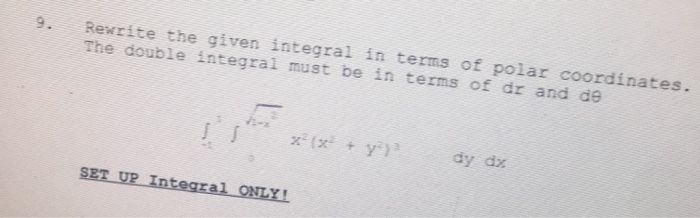Rewrite the given integral in terms of polar coordinates. The double integral must be in terms of dr and de x + y ) dy dy SET UP Integral ONLY!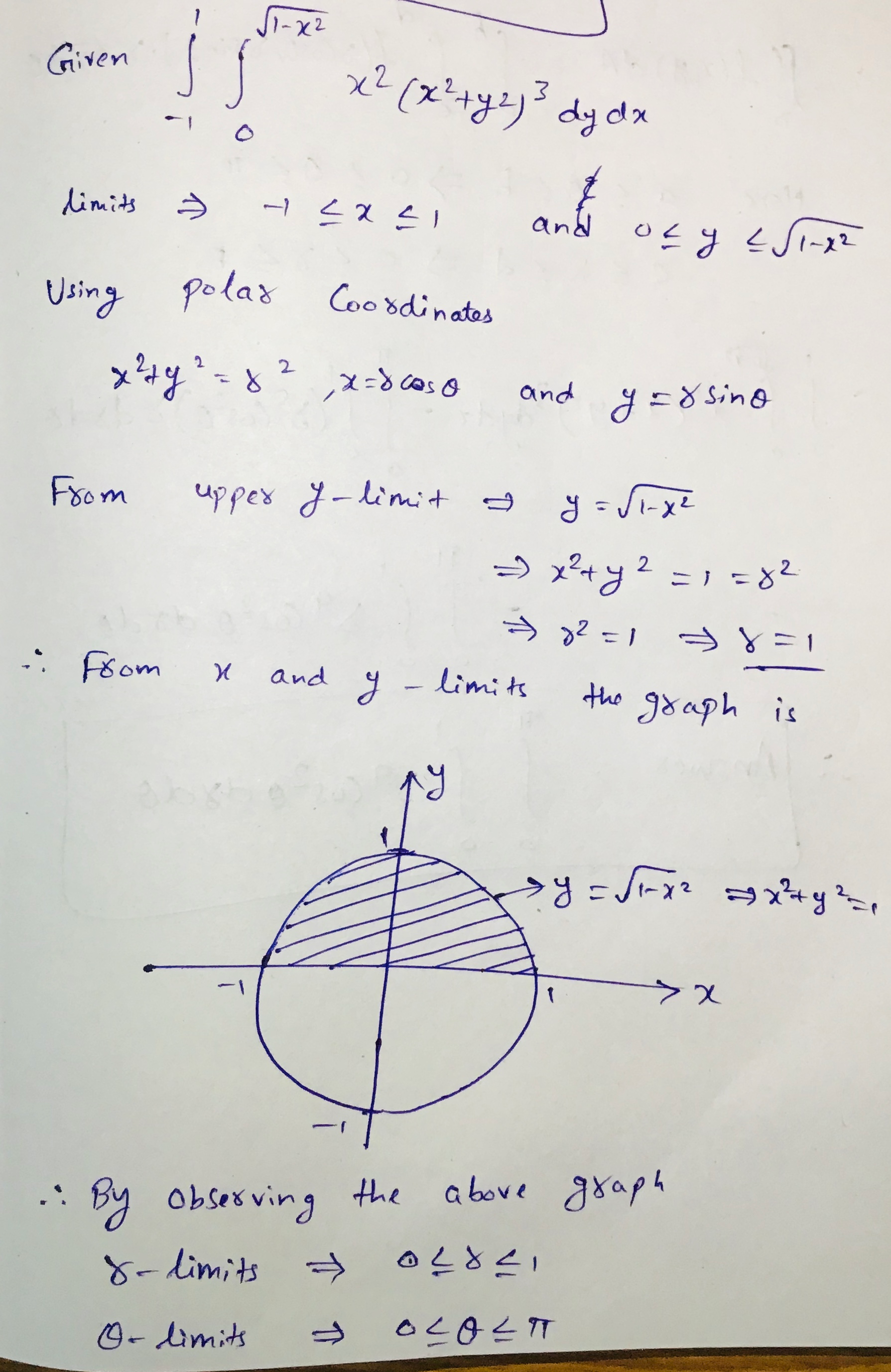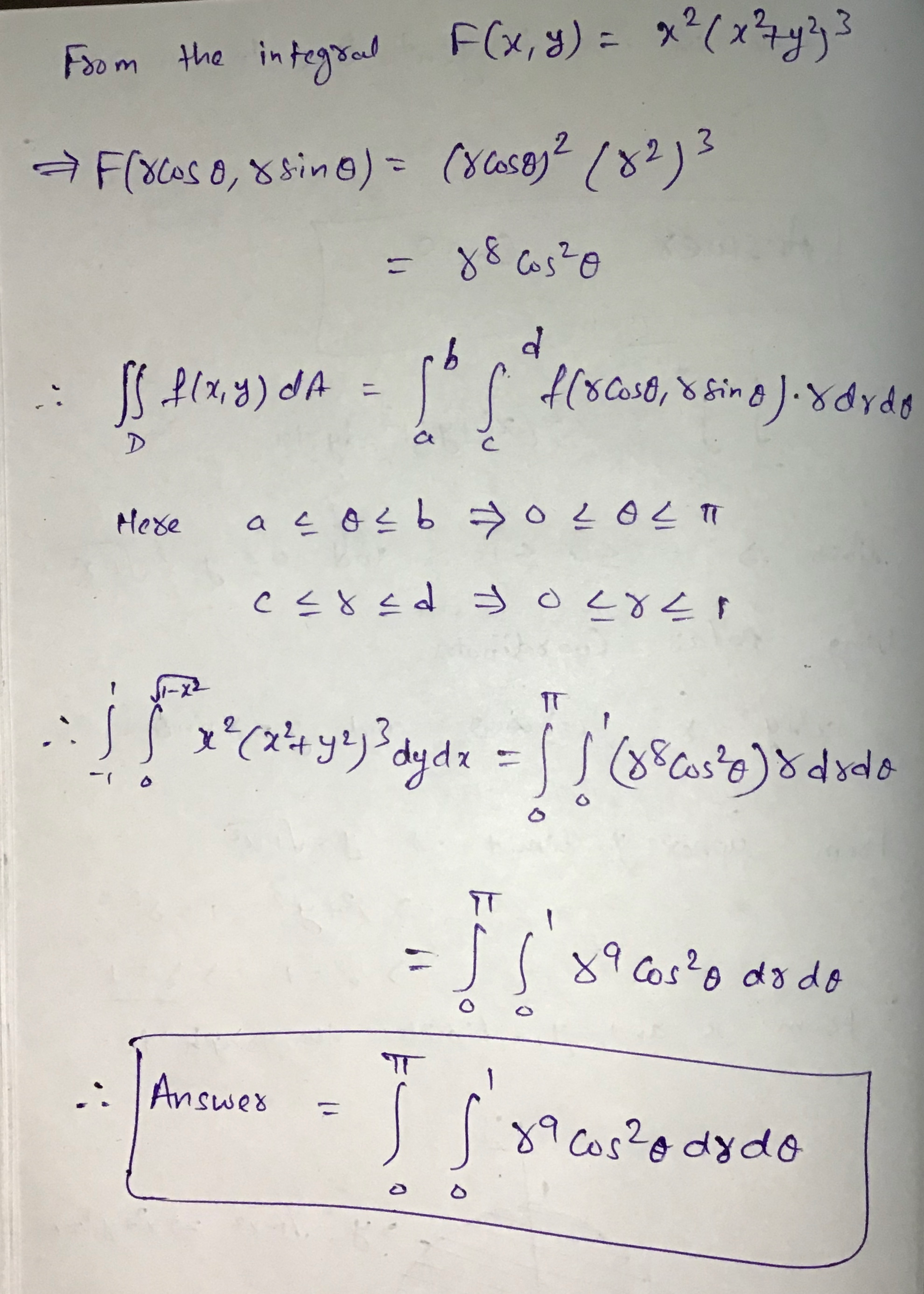#### Earn Coin

Coins can be redeemed for fabulous gifts.

Similar Homework Help Questions
• ### Using Green's Theorem, rewrite the following integral as a double integral where C is the boundary...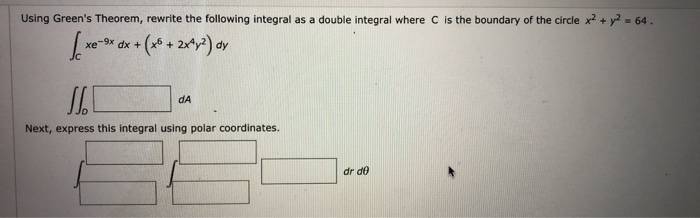Using Green's Theorem, rewrite the following integral as a double integral where C is the boundary of the circle x2 + y2 = 64. [xe-ex dx + (x + 2xy?) ay DA Next, express this integral using polar coordinates. dr de

• ### 6. (4 pts) Consider the double integral∫R(x2+y)dA=∫10∫y−y(x2+y)dxdy+∫√21∫√2−y2−√2−y2(x2+y)dxdy.(a) Sketch the region of integrationRin Figure 3.(b) By completing...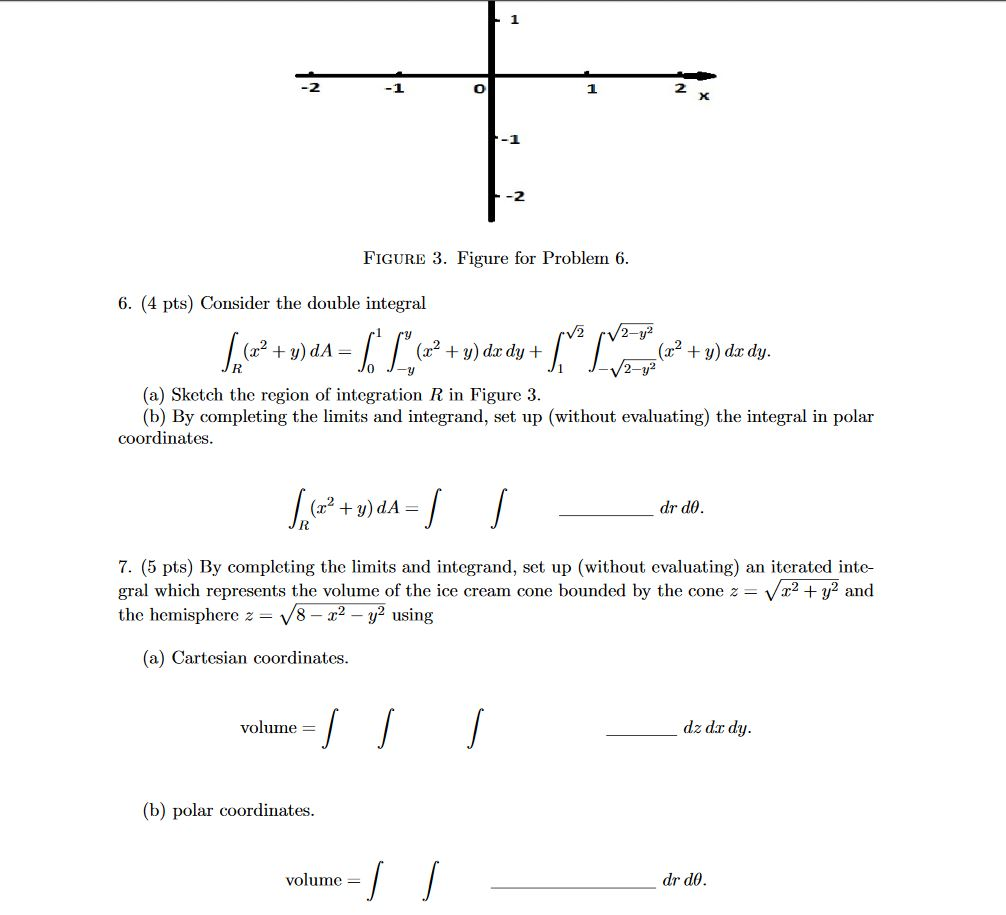6. (4 pts) Consider the double integral∫R(x2+y)dA=∫10∫y−y(x2+y)dxdy+∫√21∫√2−y2−√2−y2(x2+y)dxdy.(a) Sketch the region of integrationRin Figure 3.(b) By completing the limits and integrand, set up (without evaluating) the integral in polar coordinates. -1 -2 FIGURE 3. Figure for Problem 6. 6. (4 pts) Consider the double integral V2 /2-y² + = (x2 + y) dx dy + + y) do dy. 2-y2 (a) Sketch the region of integration R in Figure 3. (b) By completing the limits and integrand, set up (without evaluating)...

• ### Use polar coordinates to find the centroid of the following constant-density plane region The reg...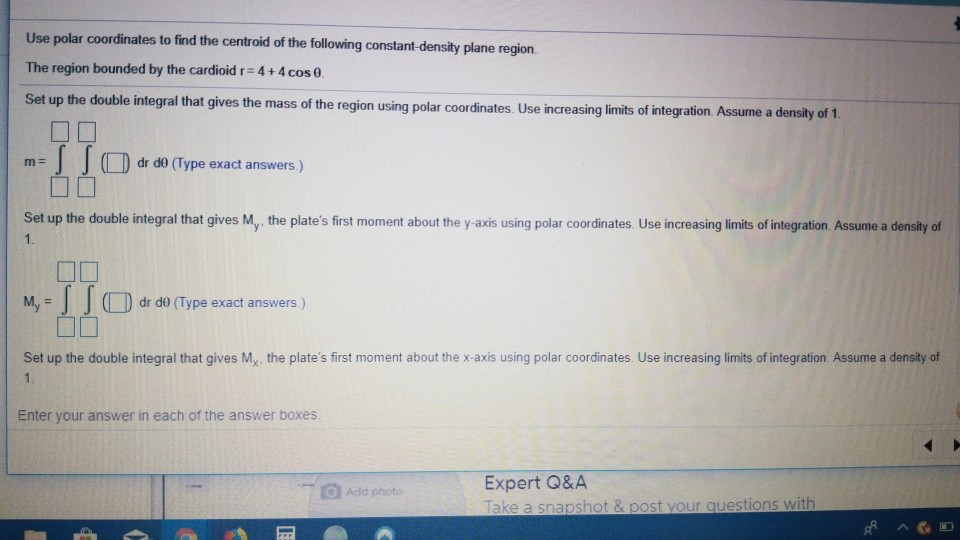Use polar coordinates to find the centroid of the following constant-density plane region The region bounded by the cardioid r4+4cos0. Set up the double integral that gives the mass of the region using polar coordinates. Use increasing limits of integration. Assume a density of 1 dr d0 (Type exact answers.) Set up the double integral that gives My the plate's first moment about the y-axis using polar coordinates. Use increasing limits of integration. Assume a density of M,-J J O...

• ### 6. (4 pts) Consider the double integral∫R(x2+y)dA=∫10∫y−y(x2+y)dxdy+∫√21∫√2−y2−√2−y2(x2+y)dxdy.(a) Sketch the region of integration R in Figure 3.(b)...6. (4 pts) Consider the double integral∫R(x2+y)dA=∫10∫y−y(x2+y)dxdy+∫√21∫√2−y2−√2−y2(x2+y)dxdy.(a) Sketch the region of integration R in Figure 3.(b) By completing the limits and integrand, set up (without evaluating) the integral in polar coordinates.∫R(x2+y)dA=∫∫drdθ.7. (5 pts) By completing the limits and integrand, set up (without evaluating) an iterated inte-gral which represents the volume of the ice cream cone bounded by the cone z=√x2+y2andthe hemisphere z=√8−x2−y2using(a) Cartesian coordinates.volume =∫∫∫dz dxdy.(b) polar coordinates.volume =∫∫drdθ. -1 -2 FIGURE 3. Figure for Problem 6. 6. (4 pts)...

• ### 6. (4 pts) Consider the double integral∫R(x2+y)dA=∫10∫y−y(x2+y)dxdy+∫√21∫√2−y2−√2−y2(x2+y)dxdy.(a) Sketch the region of integration R in Figure 3.(b)...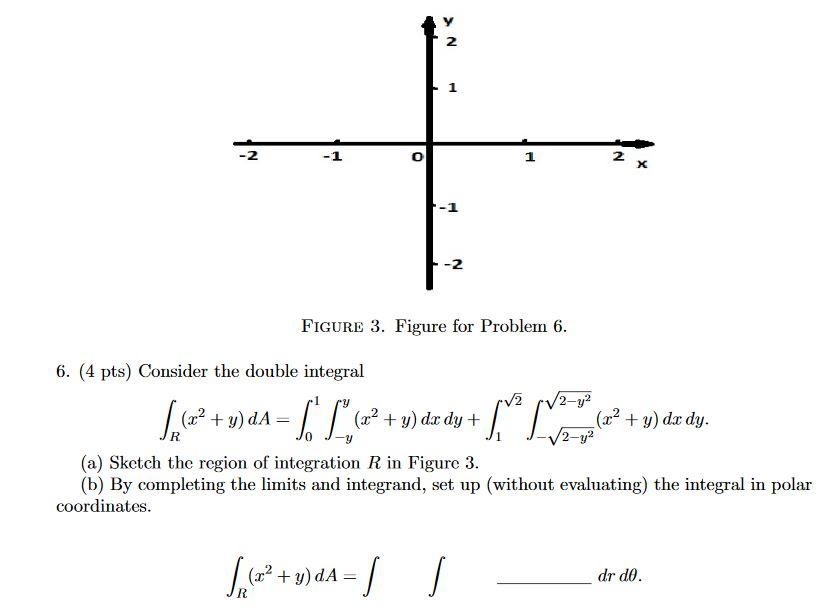6. (4 pts) Consider the double integral∫R(x2+y)dA=∫10∫y−y(x2+y)dxdy+∫√21∫√2−y2−√2−y2(x2+y)dxdy.(a) Sketch the region of integration R in Figure 3.(b) By completing the limits and integrand, set up (without evaluating) the integral in polar coordinates. 2 1 2 X -2 FIGURE 3. Figure for Problem 6. 6. (4 pts) Consider the double integral V2 2-y2 (2? + y) dA= (32 + y) dx dy + (x2 + y) dx dy. 2-y? (a) ketch the region of integration R in Figure 3. (b) By completing...

• ### Set up and evaluate the double integral using polar coordinates f(x,y) = 8-y; R is the...

Set up and evaluate the double integral using polar coordinates f(x,y) = 8-y; R is the region enclosed by the circles with polar equations r=cos(theta) and r=3cos(theta). I am struggling with understanding how to determine the interval for theta. The answer key says 0<= theta <= pi but I don't understand why. Please elaborate on this when solving.

• ### 1. Use polar coordinates to evaluate the double integral dA z2 +y where R is the...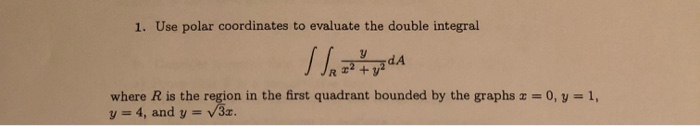1. Use polar coordinates to evaluate the double integral dA z2 +y where R is the region in the first quadrant bounded by the graphs x = 0, y = 1, y=4, and y V3z. 1. Use polar coordinates to evaluate the double integral dA z2 +y where R is the region in the first quadrant bounded by the graphs x = 0, y = 1, y=4, and y V3z.

• ### please answer both questions bex Use a double integral in Polar Coordinates to find the area...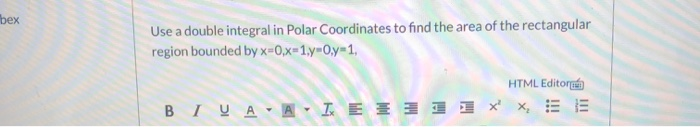please answer both questions bex Use a double integral in Polar Coordinates to find the area of the rectangular region bounded by x=0,x=1.-O.y-1, HTML Editore BIVA-AIXE 3 1 X X, SE Solve the following double integral using Polar Coordinates. x2 + y dydx HTML Editor

• ### Problem 6 [5 points] |(y ( y-dan- 0.708 Set up an equation with a double Integral in polar coordinates to find a such that the volume inside the hemisphere z 16-x-yi and outside the cylinder x2 +...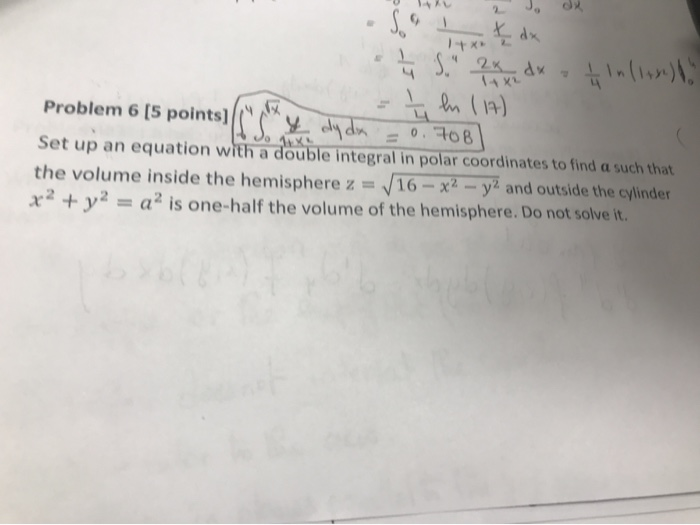Problem 6 [5 points] |(y ( y-dan- 0.708 Set up an equation with a double Integral in polar coordinates to find a such that the volume inside the hemisphere z 16-x-yi and outside the cylinder x2 + y2 = a 2 is one-half the volume of the hemisphere. Do not solve it. Problem 6 [5 points] |(y ( y-dan- 0.708 Set up an equation with a double Integral in polar coordinates to find a such that the volume inside the...

• ### polar coordinates double integrals

Evaluate the given integral by changing to polar coordinates.The integral isdouble int (x)dAwhere D is the region in the first quadrant that lies between the circles x2 + y2 = 64 and x2 + y2 = 8x.